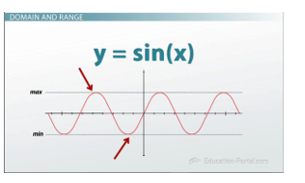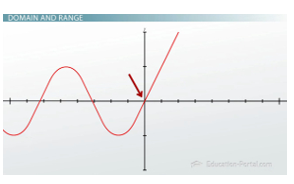# What is a Function: Basics and Key Terms

#### Video Lesson on What is a Function: Basics and Key Terms

This lesson video Not available at thisy time available video coming soon

### What is a Function: Basics and Key Terms

Mapping numbers sounds complex, but we do it when we buy gasoline. We pump gasoline, and the gas station charges us based on the amount of gas that we pump. Learn how this relates to functions while reviewing the basics and notations in this lesson.

### Basics of a Function

Would it surprise you to know that one of the most important pillars of calculus is something that you use every day? Functions are fundamental to calculus, but you have been using them your entire life. Formally, functions map a set of numbers to another set of numbers. So what does this mean?

Say we have a black box, and we're going to call this our function. If you put in the number 4, you might get out the number 8. If you put in the number 5, you might get out the number 16. For each number that you put in, say x, you'll get out another number, say y. Now sometimes you can put in two different numbers - let's say 4 and 22 - and get out the same number, say 39. But at no point in time will you put in one number and get two different numbers out. This may sound complex, but it's really just saying stuff you already know.

### Functions in our Daily Lives

For example, you use functions every time you go to the gas station. The amount of money that you pay a gas station depends on the amount of gas that you pump. Put another way, the amount of gas that you pump determines the number of dollars that you pay. Let's say gas is \$3.80 a gallon. If you're going on a long road trip, you might need 10.2 gallons. If you pump 10.2 gallons and gas is \$3.80 a gallon, the gas station is going to charge you \$38.76. If you're just running across town and only need 2.3 gallons, the gas station is only going to charge you \$8.74. Of course, depending on your car, you may need more or less gas, and the station is going charge you based on the amount of gas that you take.

How they determine the amount to charge is very simple. They use a function that maps the number of gallons that you pump to the number of dollars that you need to pay. Specifically, we say that number of dollars you pay is a function of the amount of gas you buy.

### Variables in a Function

You know the number of gallons that you're going to buy; you know an x variable. This is your independent variable. What you want to know is the amount of dollars that you're going to pay, the y variable. This is dependent, because it depends on the number of gallons that you buy. One way to put this mathematically is that the dollars you pay is a function of the gallons you buy, just like we said before. But let's write this out in math terms: The dollars you pay (y) is (that's math speak for 'equals') a function (f for function) of the gallons that you buy (x). So y= f(x). Generally, a function is written with input variables in these parentheses.

In the case of our gas station, we really know what the function is. We go to the gas station and pump some amount of gas. Because you've probably gone to the gas station before, you know that the number of dollars that you're going to pay is equal to the amount of gas that you pumped times the price per gallon. So if gas is \$4 per gallon, then we write y is equal to 4 times our input, which is the number of gallons we pump: y=4x. If we pump 4 gallons of gas, we plug this into our function, 4 * 4, and that's 16. We would owe \$16. So an important point here is that when we say y=f(x), that's good for any value of x. We're using x as a variable here. If we say y=f(5), we're evaluating this function using x=5.

Imagine going to the gas station, and instead of pumping 4 gallons of gas and owing \$16, you try to give gas back to the gas station. Now I don't know about you, but when I've tried to do that, the gas station attendant usually just laughs at me, because he and I both know that the amount of gas that I can pump (the input to our function) has to be greater than zero. The minimum amount of gas I can pump is zero, and if I go to the gas station and pump zero gallons of gas, the gas station just gets annoyed. But there is no maximum amount, practically speaking. The output of our function will be between zero and infinity.he range corresponds to the y value and the x values correspond to the domain

### Domain and Range

All possible inputs to our function is known as the domain. The domain are those values that you can put as input (as the x variable) into the function.

The output - all possible values that you can get out of your function - are known as the range. In this case, the range is the amount of dollars that you could expect to spend at the gas station.

So if I go back to the black box, we're going to write our function a little more formally now as y=f(x). The inputs to our black box are now the x values that make up the domain, and the output - something within the range - are our y values. Mathematicians will sometimes write like this, where now you've got basically two sheets of paper, in a sense, facing one another. On one side you have a domain - you have a function - and the second sheet of paper is the range.

What about the function sine of x? The function sin(x), I will write as y is some function of x and that function is actually sin(x), so y=sin(x). What about the domain and range of this function? The domain are all possible values of x that you can put into this. If you go your calculator, you know that you can put anything into sin(x), so the domain is all values from minus infinity to infinity. What about the range? Well the range is all possible values of y. If we graph sin(x), you can pretty easily see that the maximum value is going to be 1 and the minimum value is going to be -1.

So writing y=sin(x), we know that y has to be between -1 and 1. That means that the range of sin(x) is going to be between -1 and 1. So the range corresponds to the y value, this vertical axis, and the domain corresponds to the x values, this horizontal axis. One last thing: What about the domain and range of this function?Graph of the final function example

First, let's take a look at what this function means. What this means is for values of x that are less than zero, our function, f(x), equals sin(x). If I graph this out, anything that's less than zero is going to be sin(x); it's going to continue on. For values of x that are greater than zero, f(x) is going to be equal to x, so it's going to look like this. An important point to note here is that there is no value assigned at x=0, so I'm going to put a circle there because it's undefined.

### Lesson Summary

To recap, functions map some set of numbers to another set of numbers, but this is no more complex than going to the gas station. Your input to your function, your x variable, is the independent variable, and x needs to be within the domain of the function. The y variable is your dependent variable. It's the output of your function and has to be within the range of the function.

### More Educational and Fun StuffMore In-depth knowledge about what you need. Detailed about test preparation, English Writing, TOEFL, and IELTS.